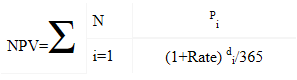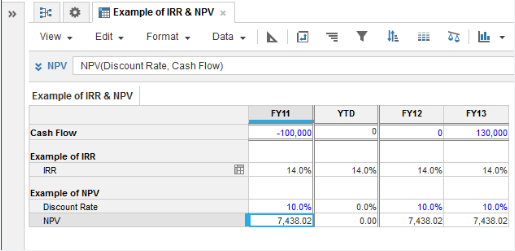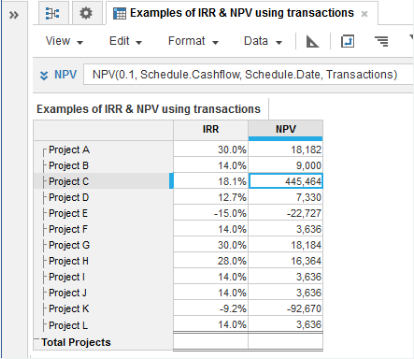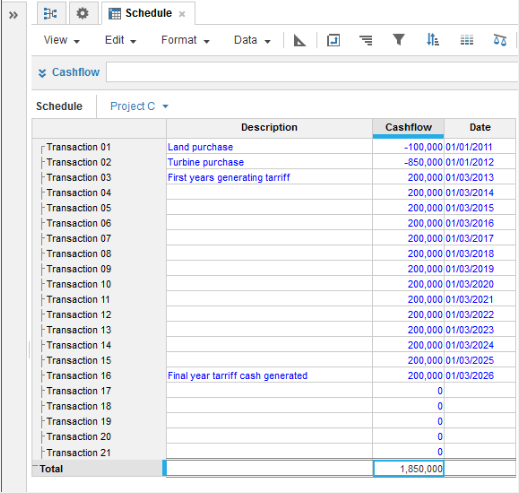1. Calculation functions
2. All Functions
3. Financial Functions
4. NPVCalculates the net present value of a series of cashflows using a constant interest rate.

If the timescale was yearly and the interest rate was 10% per annum, the syntax would be:

NPV(0.1, Cashflow)

If the rate is entered as a percentage-formatted line item, the result would be:

NPV(Rate, Cashflow)

In the latter case, the rate would be entered as 10%.

What rate to use for monthly timescales?

The rate is entered per period, so a 10% annual interest rate is equivalent to 0.7974% per month based on 12 equal periods.

 (1+r)n= 1.10 where n is the number of periods per year r = ((1.10) 1/12) - 1 = 1.007974 or 0.7974%

Whatever timescale is used for the cashflow, the NPV result is a single solution that does not require a timescale dimension. The NPV result is calculated based on cash flows over the entire span of the timescale.

NPV is the solution to the equation shown:• Rate is the discount rate.
• di is the number of days from the start of the first time period
• Pi is the payment in the ith period.
• N is the number of payments in and out over the entire timescale.

## Syntax

NPV(d, c, [m, t]) where:

• d: Number: Discount Rate. Enter as a number, such as 0.1 meaning 10%
• c: Number: Cash flow. Must have at least one negative and one positive value
• m: The date of each transaction
• t: The name of the list containing the schedule of transactions

## Format

Input Format Output Format

d: Number: percentage

c: Number: Decimal

m: The date of each transaction

t: The name of the list containing the schedule of transactions

Number

## Arguments

The function uses the following arguments:

• d: Number: Numeric line item, property, or expression
• c: Number: Numeric line item, property, or expression
• m: Date: Hard-coded date, date variable, or line item
• t: Transaction List: List-formatted line item

### Use NPV with the Users list

You can reference the Users list with the NPV function. However, you cannot reference specific users within the Users list as this is production data, which can change and make your formula invalid.

## Constraints

The function has no constraints.

## Example#### NPV using Dates

NPV(Discount Rate, Cashflow, Date, Transactions)
• Discount Rate: Enter as a number, such as 0.1 meaning 10%. Alternatively input into a percentage-formatted variable in the module itself.
• Cashflow: Numeric cash values. Must have at least one negative and one positive value.
• Date: The date of each transaction
• Transactions: The name of the list containing the schedule of transactions.
The NPV result itself does not require a time dimension.

NPV Example using Dates

In the example shown, a series of cash transactions and the dates are entered in the module named Schedule. This module has a list named Transactions as one of its dimensions. The IRR and NPV are calculated for each project.If you're using the NPV function in this way, the first transaction in the series must have a Cashflow value and a Date entered.